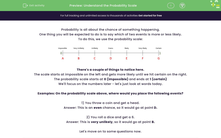# Understand the Probability Scale

In this worksheet, students will practise placing probabilities on a scale.Key stage:  KS 4

Year:  GCSE

GCSE Subjects:   Maths

GCSE Boards:   AQA, Eduqas, Pearson Edexcel, OCR,

Curriculum topic:   Probability

Curriculum subtopic:   Probability Basic Probability and Experiments

Difficulty level:#### Worksheet Overview

Probability is all about the chance of something happening.

One thing you will be expected to do is to say which of two events is more or less likely.

To do this, we use the probability scale:There's a couple of things to notice here.

The scale starts at impossible on the left and gets more likely until we hit certain on the right.

The probability scale starts at 0 (impossible) and ends at 1 (certain)

We'll focus on the numbers later - let's just look at words today.

Examples: On the probability scale above, where would you place the following events?

1) You throw a coin and get a head.

Answer: This is an even chance, so it would go at point D.

2) You roll a dice and get a 6.

Answer: This is very unlikely, so it would go at point B.

Let's move on to some questions now.

### What is EdPlace?

We're your National Curriculum aligned online education content provider helping each child succeed in English, maths and science from year 1 to GCSE. With an EdPlace account you’ll be able to track and measure progress, helping each child achieve their best. We build confidence and attainment by personalising each child’s learning at a level that suits them.

Get started